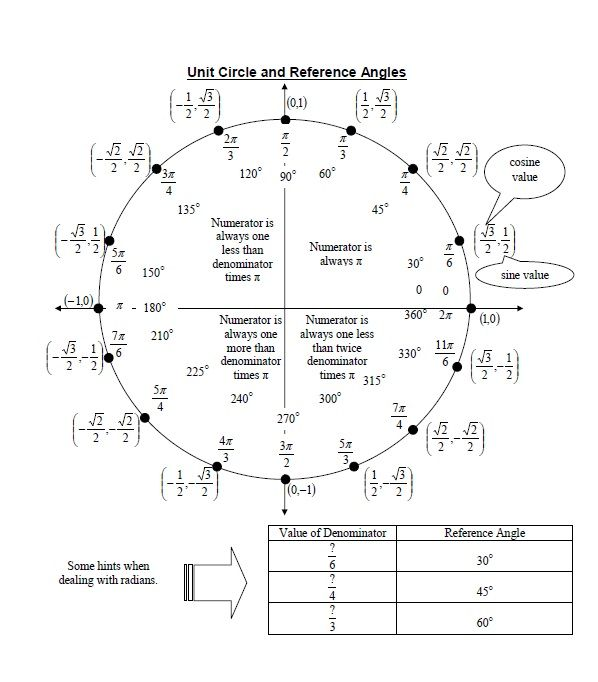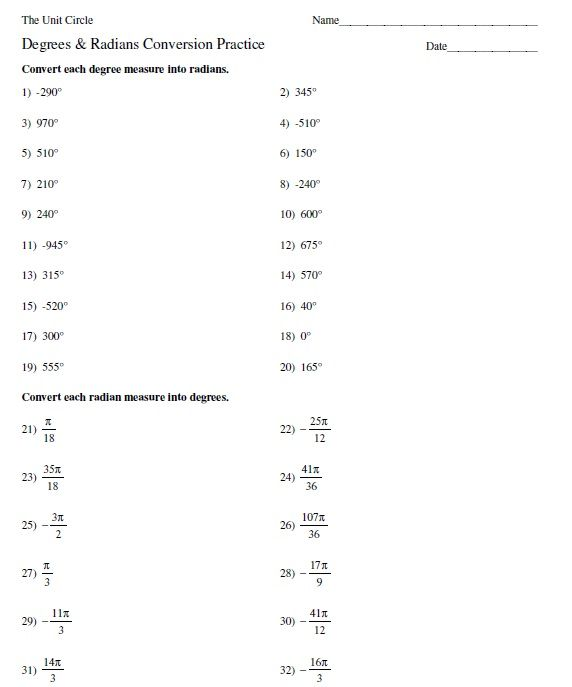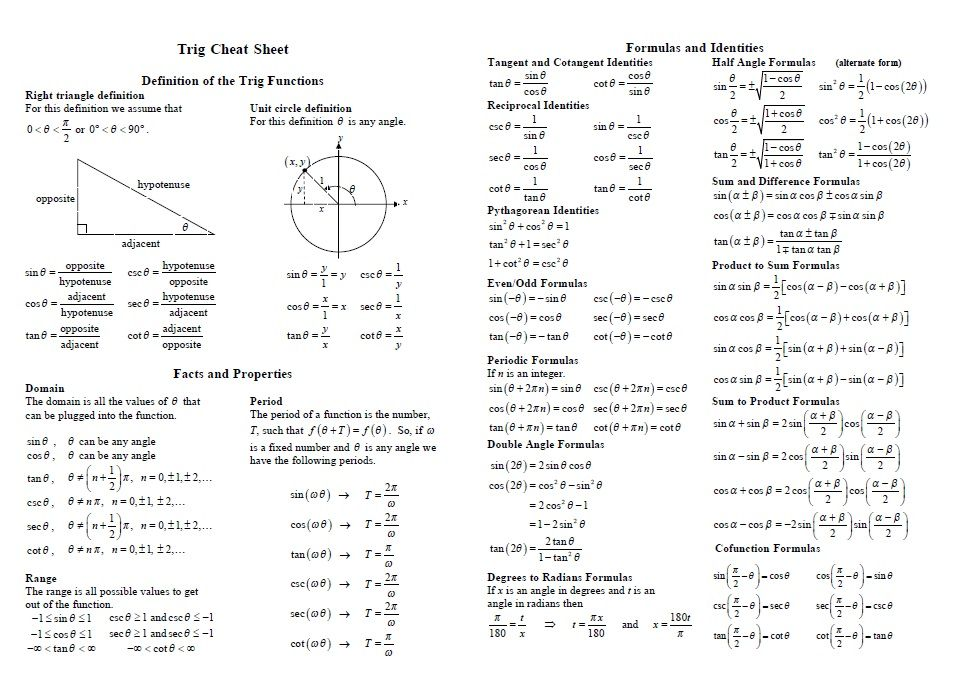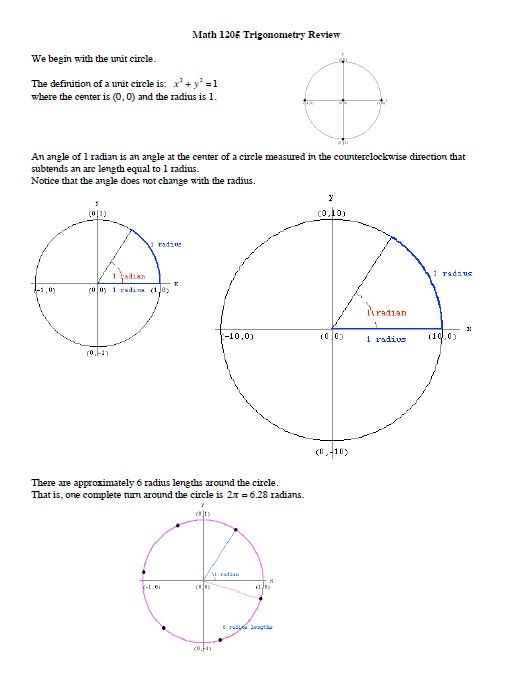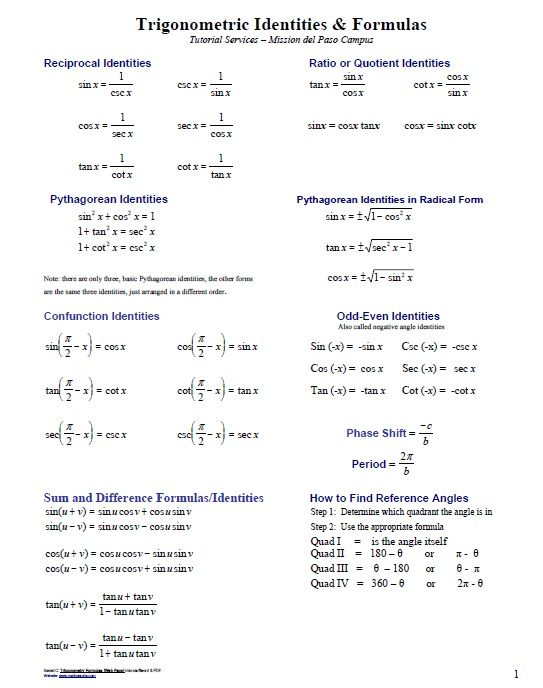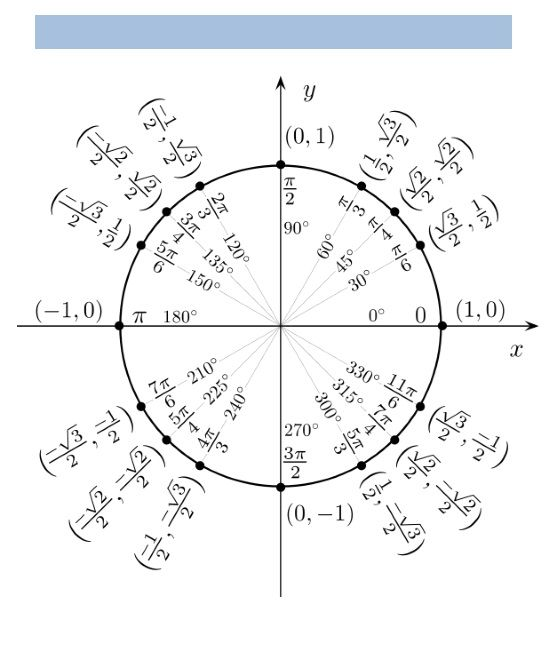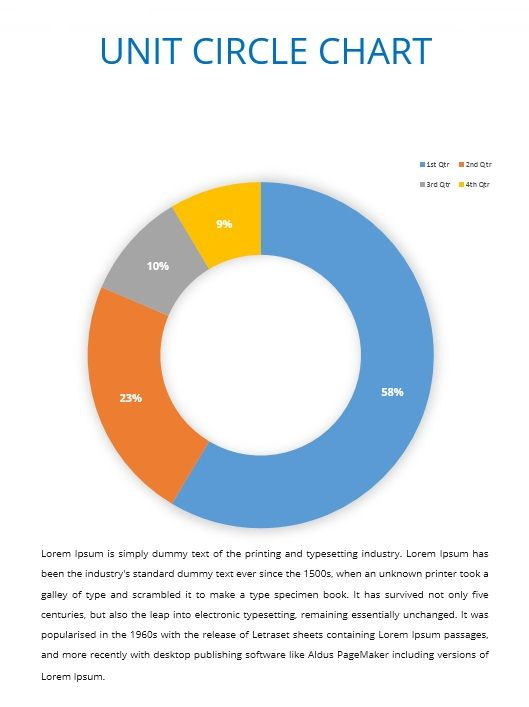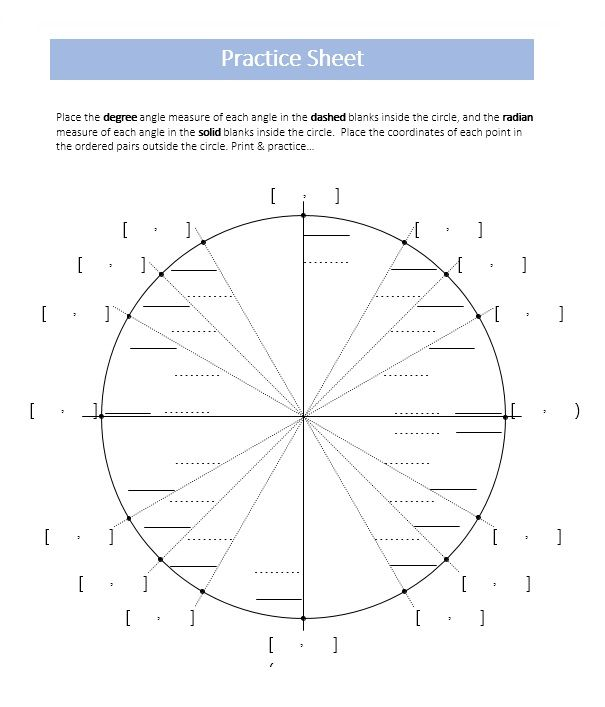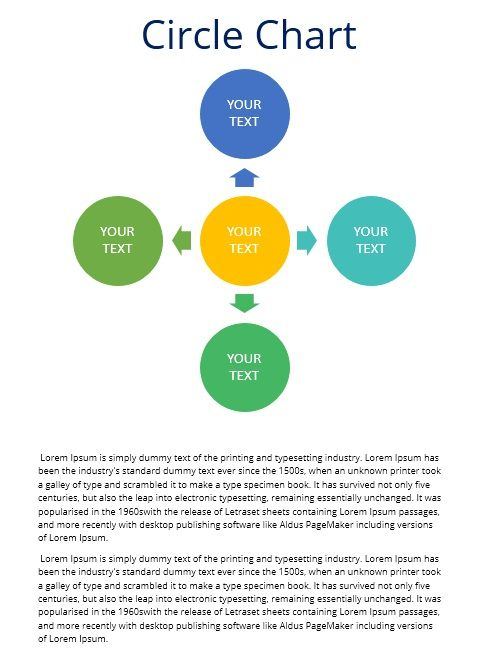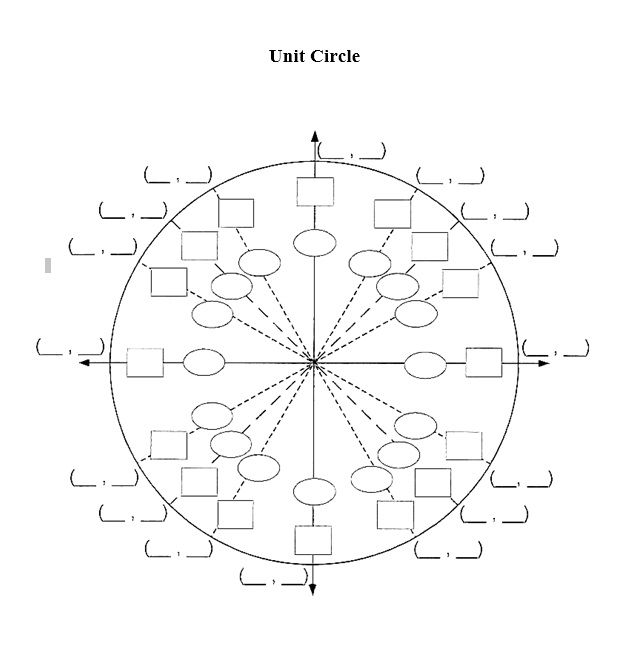# 15+ Free Unit Circle Charts

Download these 15+ Free Printable Unit Circle Charts & Diagrams in MS Word as well as in PDF Format.

Trigonometry is a complex subject for most of the students but some find it interesting and fascinating. Although the geometric equations and theorems may seem complicated, but when you have the basic understanding and you find out the building blocks or foundation of a theorem, it seems not so complicated at all. Unit circle is one of the many parts that together make up trigonometry. As the name suggests; a unit circle is a circle with unit or 1 radius. In simpler words, the diameter of this circle is 2 as diameter is equal to twice the radius. You can also understand with the fact that when a line is drawn from the center of the circle to any point on the edge of the circle, the radius will be 1. The center point is what we call the origin or the point where x-axis and y-axis meet together.

The most basic purpose of using a unit circle or what we commonly refer to as trigonometry circle is that it allows us to calculate the tangent, cosine and sine of any angle that stays in between 0 degree to 360 degrees. If you draw a horizontal line that is also known as cosine to the edge of the circle and then draw a vertical line from the origin as well and it will be known as sine. These two lines will form a triangle with a specific angle and you can solve this triangle using Pythagorean Theorem that states that 2 sides of a triangle are equal to the length of the third side.

## Free Unit Circle Charts

Here are several free Unit Circle Charts that you can download to study and understand it.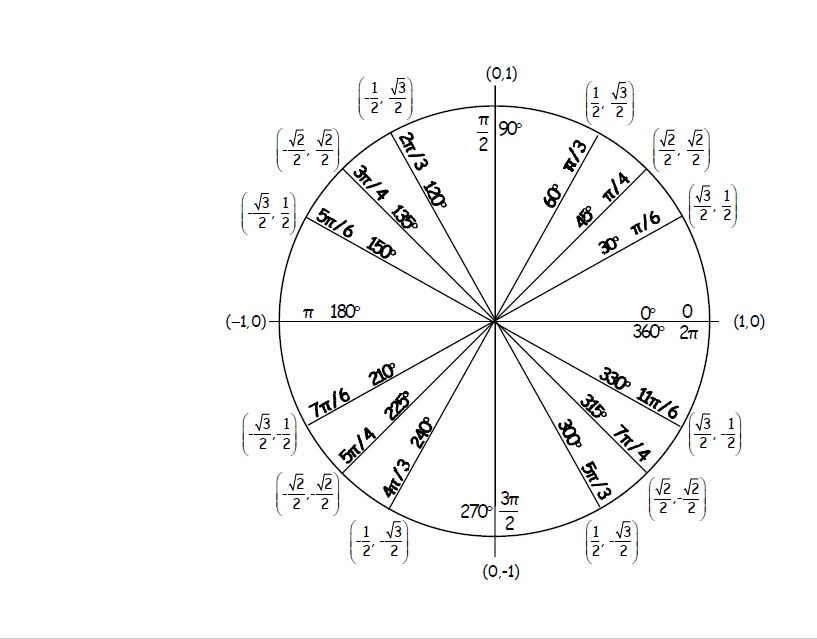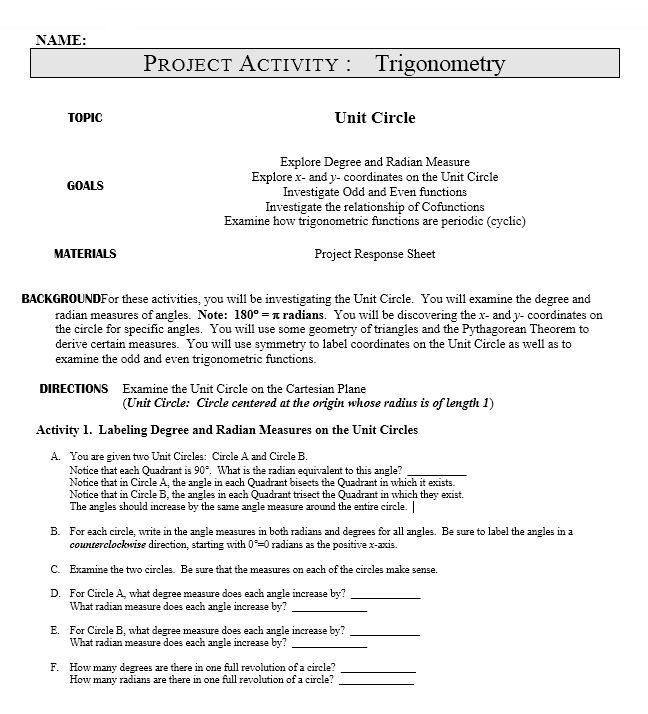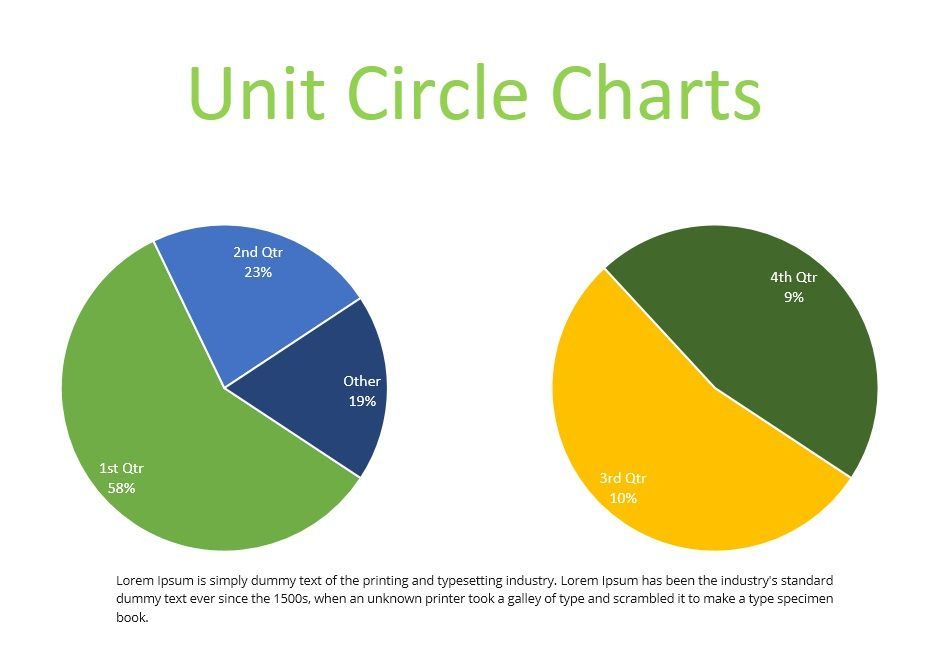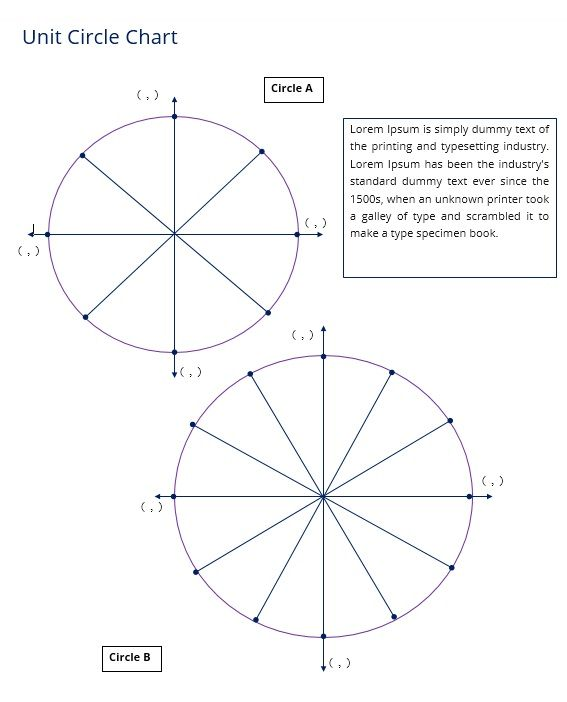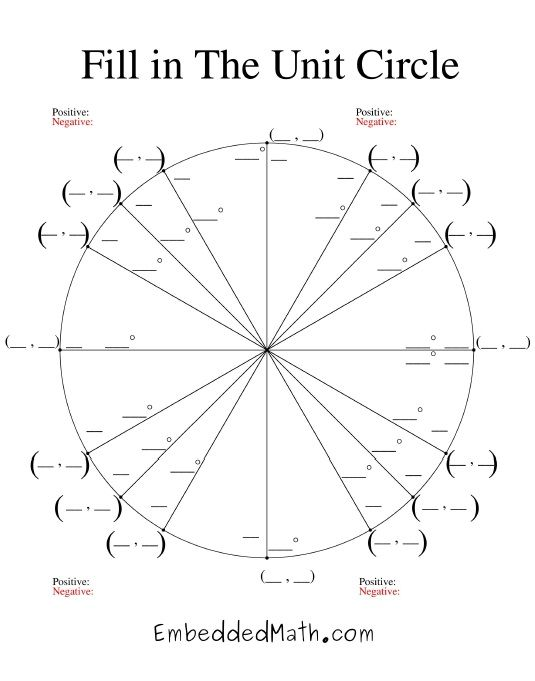source: EmbeddedMath.com